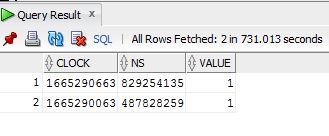# Oracle DB slow query when rownum is using

XMLWordPrintable

#### Details

•Problem report
• Status: Closed
•Trivial
• Resolution: Fixed
• None
• None
• Team A
• Sprint 95 (Dec 2022), Sprint 96 (Jan 2023)
• 0.25

#### Description

Oracle 19.1.0 with partitioning with internal jobs and procedures. DB host performance and health is fine. There is no load for disks or CPU/memory.

Zabbix Server 5.0.24 with Proxy. For now all Proxy are disabled.

Main issue - history_uint related queries extremely slow. We cannot even get get any last data or graphs. Important point here - trends work fine. We can get graphs for more then 5 day period, when trends is using.

Slow queries is mostly this one:

```1529  19:43:02.781010 write(8, "  1529:20221010:194302.780 slow query: 587.294631 sec, \"select * from (select clock,ns,value from history_uint where itemid=3290659 and clock<=1665312259 and clock>1664707459 order by clock desc) where rownum<=2\"\n", 213) = 213 <0.000013>
Line 353: 1529  19:43:02.781010 write(8, "  1529:20221010:194302.780 slow query: 587.294631 sec, \"select * from (select clock,ns,value from history_uint where itemid=3290659 and clock<=1665312259 and clock>1664707459 order by clock desc) where rownum<=2\"\n", 213) = 213 <0.000013>
Line 373: 1519  19:43:02.781607 write(8, "  1519:20221010:194302.781 slow query: 587.259824 sec, \"select * from (select clock,ns,value from history_uint where itemid=3607148 and clock<=1665312248 and clock>1664707448 order by clock desc) where rownum<=1\"\n", 213) = 213 <0.000027>
Line 373: 1519  19:43:02.781607 write(8, "  1519:20221010:194302.781 slow query: 587.259824 sec, \"select * from (select clock,ns,value from history_uint where itemid=3607148 and clock<=1665312248 and clock>1664707448 order by clock desc) where rownum<=1\"\n", 213) = 213 <0.000027>
Line 395: 1504  19:43:02.782209 write(8, "  1504:20221010:194302.781 slow query: 587.079826 sec, \"select * from (select clock,ns,value from history_uint where itemid=4863552 and clock<=1665312253 and clock>1664707453 order by clock desc) where rownum<=2\"\n", 213) = 213 <0.000016>
Line 395: 1504  19:43:02.782209 write(8, "  1504:20221010:194302.781 slow query: 587.079826 sec, \"select * from (select clock,ns,value from history_uint where itemid=4863552 and clock<=1665312253 and clock>1664707453 order by clock desc) where rownum<=2\"\n", 213) = 213 <0.000016>
Line 740: 1505  19:44:51.229668 write(8, "  1505:20221010:194451.229 slow query: 671.026438 sec, \"select * from (select clock,ns,value from history_uint where itemid=1128817 and clock<=1665312257 and clock>1664707457 order by clock desc) where rownum<=3\"\n", 213) = 213 <0.000016>
Line 740: 1505  19:44:51.229668 write(8, "  1505:20221010:194451.229 slow query: 671.026438 sec, \"select * from (select clock,ns,value from history_uint where itemid=1128817 and clock<=1665312257 and clock>1664707457 order by clock desc) where rownum<=3\"\n", 213) = 213 <0.000016>
Line 754: 1531  19:44:51.235144 write(8, "  1531:20221010:194451.235 slow query: 567.998976 sec, \"select * from (select clock,ns,value from history_uint where itemid=2915063 and clock<=1665312263 and clock>1664707463 order by clock desc) where rownum<=2\"\n", 213) = 213 <0.000015>
Line 754: 1531  19:44:51.235144 write(8, "  1531:20221010:194451.235 slow query: 567.998976 sec, \"select * from (select clock,ns,value from history_uint where itemid=2915063 and clock<=1665312263 and clock>1664707463 order by clock desc) where rownum<=2\"\n", 213) = 213 <0.000015> ```

Where select * from (select . . .  ) where rownum<=2 is using.

Query without rownum take seconds:But with:

`select * from (select clock,ns,value from history_uint where itemid=2915063 and clock<=1663707463 and clock>1663501938 order by clock desc) where rownum<=2;`From slow query plan we can see that STOPKEY is using for such queries.

This issue is very similar to slow top-n.
http://www.dba-oracle.com/t_sql_tuning_rownum_equals_one.htm

#### PeopleVladislavs SokurenkoAleksey Volodin